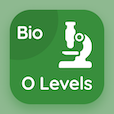Class 9 Online Courses

Grade 9 Math MCQs PDF - Topics

# Binary Relation MCQ Quiz Online

Practice Binary relation Multiple Choice Questions (MCQ) to solve binary relation quiz answers PDF to learn 9th grade math online course. Sets and Functions Multiple Choice Questions and Answers (MCQs), Binary Relation quiz questions PDF for online degrees. "Binary Relation MCQ" PDF Book: grade 9 math problems, sets, ordered pairs test prep for online secondary school courses.

"The set consisting of all the second elements of each ordered pair in the relation is called" Multiple Choice Questions (MCQ) on binary relation with choices domain of relation, range of relation, subset, and complement of a set for online degrees. Learn sets and functions quiz questions for online certificate programs for online teaching certification programs.

## MCQs on Binary Relation

MCQ: The set consisting of all the second elements of each ordered pair in the relation is called

domain of relation
range of relation
subset
Complement of a set

MCQ: The range of R = {(0, 2), (2, 4), (3, 4), (4, 5)} is

{0, 2, 4, 5}
{0, 2, 3, 4}
{2, 4, 5}
{3, 4, 5}

MCQ: The set consisting of all the first elements of each ordered pair in the relation is called

subset
domain of relation
range of relation
Complement of a set

MCQ: The domain of R = {(0, 2), (2, 4), (3, 4), (4, 5)} is

{0, 2, 4, 5}
{0, 2, 3, 4}
{2, 4, 5}
{3, 4, 5}

### More Topics from Grade 9 Math Course